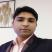# Mensuration: Overview, Questions, Preparation

Mensuration 2021 ( Mensuration )

2.3K ViewsRachit Kumar SaxenaManager-Editorial

1. What is Mensuration?
2. Weightage and Importance of Mensuration
3. Illustrated example on Mensuration
4. FAQs on Mensuration

## What is Mensuration?

Mensuration is the measurement of geometric figures. This chapter will learn about some additional quadrilaterals, their areas, perimeter, surface areas, and volumes.

### Trapezium:

A trapezium is outlined by a form that has 2 parallel sides, and the other 2 are nonparallel. It has four sides and four vertices.Shape

Area

Perimeter

1.    Trapezium

Area= (1/2) H + (AB+CD)

P= sum of all the sides i.e. AB+BC+CD+AD

Area= Area of △ABC + Area of △ADC

The perimeter depends on the type of shape which is present

3.    Special quadrilateral (rhombus)

½ × d1 × d2

P=4a

Where, H= height, W= breadth, a,b,c,d= sides, d= diagonalsSurface area: The definition is that the total area of all the surfaces of any form.
Volume: the degree is outlined by the number any third-dimensional form takes.Solid shapes:
Solid shapes are measured by their three-dimensional structure with outlined breadth, height and length. A cube, sphere, cylinder, cuboid are solid shape examples.Shape

Surface area

Volume

1.    Cube

Area= 6a^2

V= a^3

2.    Cuboid

Area= 2 [(l × b) + (l× h) + (b× h)]

V= l*w*h

3.    Cylinder

Ares= 2πr (r + h)

V= πr2 × h

Where, H= height, a= sides, r= radius, d= diameter, b= breadth, w= width

## Weightage and Importance of Mensuration

The chapter Mensuration is in class 8. It is chapter 11 of NCERT textbook. The weightage is 15% of the total marks.

## Illustrated example on Mensuration

1. Calculate the area of a rhombus whose diagonals 10 cm and 8.2 cm of length.
Solution:

The formula for calculating area of a rhombus = ½ d1d2, (d1 and d2 are the lengths of the diagonal)
a= 2 × 10 × 8.2 cm2 = 41 cm2.

2. A rhombus with an area of 240cm2 and whose one diagonal is of length 16 cm. Find the other diagonal.

Solution:

We know d1 = 16 cm and let the other diagonal = d2

Thus the area of the rhombus = 2 d1 . d2 = 240,

So, ½ 16 d2 = 240

Therefore, d2= 30cm.

Diagonal 2 is 30 cm.

3. A cuboid-shaped aquarium is a present whose external measurements are of 80 cm × 30 cm × 40 cm. The base, side faces and back face are to be covered with a coloured paper. Find the area of the paper needed?

Solution: The length of the aquarium = l = 80 cm

Width of the aquarium  (b) = 30 cm

Height of the aquarium  (h) = 40 cm

Thus the area of the base = 80 × 30 = 2400 cm2

Area of the side face = 30 × 40 = 1200 cm2 &

Area of the back face = 80 × 40 = 3200 cm2

Thus area required to be calculated = Area of the base + area of the back face + (2 × area of a side face)
=  2400 + 3200 + (2 × 1200) = 8000 cm2

## FAQs on Mensuration

Q: What is the formula of the area of a cube?

A: The Formula for area of sq. can be:
Area=6a^2
Where a= sides of the cube

Q: What is the area of the quadrilateral?

A: The area of the quadrilateral depends on the form of that specific quadrilateral.
Example: area of general quadrilateral= space of △ABC + Area of △ADCQ: What is the height of a cylindrical structure?

A: The height of a cylinder is calculated by multiplying the world of the cylinder’s base (the circle) with its height.

Q: How many edges and faces will a cuboid have?

A: A cuboid has vi faces, 12 edges.

Q: What is the area of a cuboid?

A: Surface area= Area= two [(l × b) + (l× h) + (b× h)]
Where, l= length, h=height, b= breadth